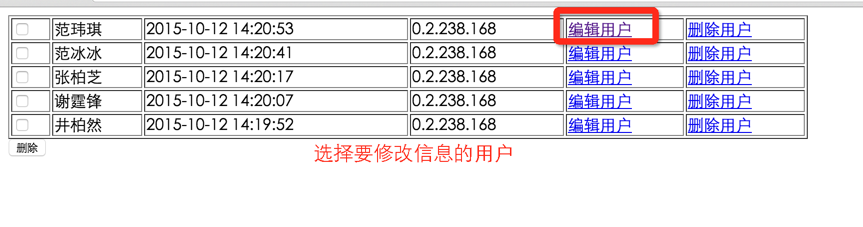• 注册

# php数据库操作之修改用户信息edit.php展示用户信息

```<?php

if (is_numeric(\$_GET['id'])) {

\$id = (int) \$_GET['id'];

}

\$sql = "select id,username from user where id = " . \$id;

\$result = mysqli_query(\$conn, \$sql);

\$data = mysqli_fetch_assoc(\$result);

?>```

```<form action="update.php" method="post">
<input type="hidden" value="<?php echo \$data['id'];?>" name="id" />
<input type="submit" value="提交">
</form>```

update.php修改操作用户数据

1.用户修改了密码

2.用户没有修改密码

1.如果他没有修改密码，也给他提示成功

2.如果他修改了用户的密码。我们真正的修改掉用户的密码，也提示修改成功。

```\$id = (int)\$_GET['id'];

```\$sql = "update user set password='" . \$password . "' where id = \$id";
\$result = mysqli_query(\$conn, \$sql);
if (\$result) {
echo '修改成功';
}```

```<?php

if (is_numeric(\$_GET['id'])) {

\$id = (int) \$_GET['id'];

}

\$sql = "select id,username from user where id = " . \$id;

\$result = mysqli_query(\$conn, \$sql);

\$data = mysqli_fetch_assoc(\$result);

?>

<form action="update.php" method="post">

<input type="hidden" value="<?php echo \$data['id'];?>" name="id" />

<input type="submit" value="提交">

</form>
<?php

mysqli_close(\$conn);

?>```

update.php修改的源代码

```<?php
include 'connection.php';

\$id = (int) \$_POST['id'];

\$sql = "update user set password='" . \$password . "' where id = \$id";

} else {

echo '修改成功';
}

\$result = mysqli_query(\$conn, \$sql);

if (\$result) {
echo '修改成功';
}```

||
<?php include 'connection.php'; \$id = (int) \$_POST['id']; if (trim(\$_POST['password'])) { \$password = md5(trim(\$_POST['password'])); \$sql = "update user set password='" . \$password . "' where id = \$id"; } else { echo '修改成功'; } \$result = mysqli_query(\$conn, \$sql); if (\$result) { echo '修改成功'; } ?>• 推荐课程
• 评论
• 问答
• 笔记
• 课件下载

phpcn_u84239

edit.php没有连接数据库，用之前的connection.php即可：include 'connection.php';

4年前    添加回复 0

4年前 0

4年前 0

4年前 0

echo '<tr> <td colspan="6"> <a href="list.php?page=1">首页</a> <a href="list.php?page=' . (\$page - 1) . '">上一页</a> <a href="list.php?page=' . (\$page + 1) . '">下一页</a> <a href="list.php?page=' . \$total . '">尾页</a> 当前是第 ' . \$page . '页 共' . \$total . '页 共'.\$count.'条 </td> </tr>'; echo '</table>'; }else{ echo '暂无数据<br>'; } mysqli_close(\$conn); ?>

4年前 0

\$result=mysqli_query(\$conn,\$sql); if(\$result&&mysqli_num_rows(\$result)){ // echo '<table width="800" border="1">'; echo '<th></th>'; echo '<th>用户名</th>'; echo '<th>密码</th>'; echo '<th>注册时间</th>'; echo '<th>注册ip</th>'; echo '<th>操作</th>'; while(\$row=mysqli_fetch_assoc(\$result)){ echo '<tr>'; echo '<td><input type="checkbox" name="id[]" value="' . \$row['id'] . '" /></td>'; echo '<td>'.\$row['username'].'</td>'; echo '<td>'.\$row['password'].'</td>'; echo '<td>'.\$row['createtime'].'</td>'; echo '<td>'.\$row['createip'].'</td>'; echo '<td> <a href="edit.html?id='.\$row['id'].'">编辑</a>&nbsp;&nbsp; <a href="delete.php?id='.\$row['id'].'">删除</a></td>'; echo '</tr>'; }

4年前 0

4年前 0

4年前 0

4年前 0

4年前 0